• Save

# Time and Speed Formula for Competitive Exams

Last year 4.2K Views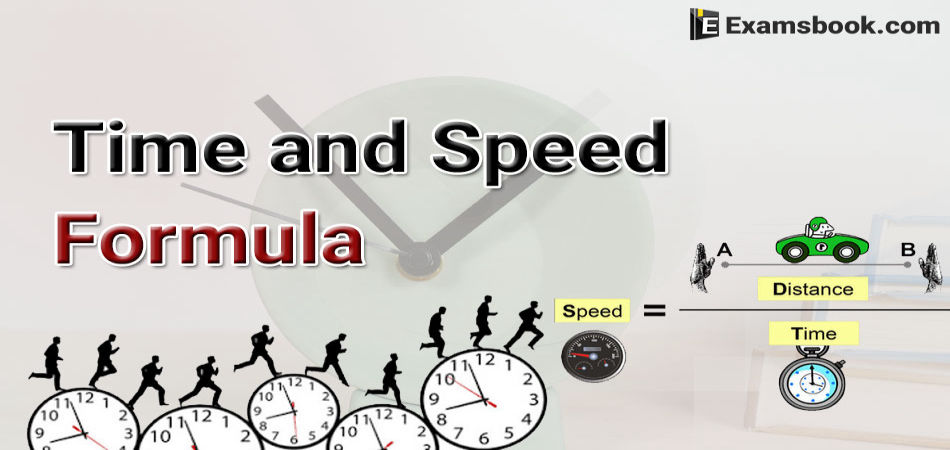If you want to get a good rank in IBPS PO, IBPS Clerk, SBI PO, SBI Clerk, SSC, CGL, SSC, CHSL, and other competitive exams, then you should pay attention to the subject of time, distance and speed. Although this topic may be a bit daunting, if you pay attention to distance, time and speed formula, then you can solve the answer to this topic easily.

The formula given here will help you solve the time and speed questions in the exam. So you should practice these sources continuously and should more practice with objective type time and speed problems for better preparation.

## Important Time and Speed Formulas

Speed

Speed is defined as the distance covered per unit time. It is the rate at which the distance is covered.

Hence, Speed =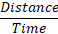Distance

When an object is moving with a certain speed in a particular time, the displacement made by an object is called the distance.

Hence, Distance = Speed×Time

Time

Time is defined as quantity, which governs the order or sequence of an occurrence. In the absence of time, the actual sequence of any occurrence or incident would be lost. If we did not have the concept of time, we would not be able to know in what period or in what order something took place.

Hence, Time =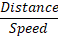Keep In Mind

(A) x km/h =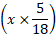m/s and

(B) y m/s =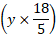km/h

(C) If the ratio of speed of A and B is x: y, then the ratio of time taken by them to cover same distance is given by

$${1\over x}: {1\over y}\ i.e\ y : x$$

Time Speed And Distance Formula With Example.

Q.1. Ashutosh covers a certain distance between his home and college by cycle. Having an average speed of 30 km/h, he is late by 20 min. However, with a speed of 40 km/h, he reaches his college 10 min earlier. Find the distance between his house and collage.

(A) 60 km

(B) 50 km

(C) 40 km

(D) 20 km

When a person covers a certain distance between two certain place with speed ‘a’, he gets his destination late by time t1 but when he covers the same distance with speed ‘b’. He reaches his destination t2 time earlier. In this case, the distance between two places is given by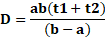Solution:

According to the formula, a = 30, b = 40

t1= 20/60, t2= 10/60

⸫ Required Distance =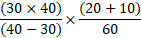=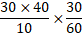= (30×4)×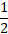= 60 km

Q.2. A Motorcycle move ate the speed of 80 km/hr. What is the speed of the car in meters per second?

(A) 8 m/sec

(B)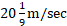(C) 15 m/sec

(D) None of these

Solution

km/hr to m/sec conversion

X km /hr =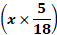m/sec.

According to the formula,

Speed =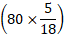m/sec = 200/9 m/sec  =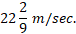Q.3. From stations M and N, two trains start moving towards each other at speed 125 km/h and 75 km/h respectively. When the two trains meet each

(A) 190 km

(B) 200 km

(C) 145 km

(D) 225 km

Solution

Given, a = 125 km/h, b = 75 km/h d = 50 km

Now, distance between the stations M and N

$$= {a+b\over a-b}×d$$

$$\left({125+75\over 125-75}\right)×50$$

$$= {200\over 50}×50=200$$

Q.4. A train passes two persons who are walking in the direction opposite to the direction of train at the rate of 10 m/s and 20 m/s respectively in 12s and 10s respectively. Find the length of the train.

(A) 500 m

(B) 900 m

(C) 400 m

(D) 600 m

Solution

Given, T1 = 12s, T2 = 10s,

a = 10 m/s and b = 20 m/s

Now, length of the train

$$= {Difference\ in \ speeds × T1 × T2\over T1- T2}$$

$$= {(20-10)×12×10}\over 12-10$$

$$={(10×12×10)\over 2} = 600$$

Q.5. Two friends X and Y walk from A to B at distance of 39 km, at 3 km an hour and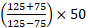km an hour respectively Y reaches B, returns immediately and meet X at C. Find the distance from A to C.

(A) 26 s

(B) 25 s

(C) 15 s

(D) 27 s

Solution

Relative speed = (84+60) km/h

= 144 km/h

$$=144×{5\over 18}m/sec = 8×5 = 40 \ m/s$$

$$Distance \ covered \ in \ passing \ each \ other = (512+528) m = 1040 m$$

$$⸫ \ Required \ time\ = {1040\over 40} = 26s.$$